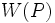# Conjugacy functor that controls fusion

Jump to: navigation, search
This article defines a property that can be evaluated for a conjugacy functor on a finite group. |View all such properties

## Definition

Suppose$G$ is a finite group and$p$ is a prime number. Suppose$W$ is a conjugacy functor on the nontrivial$p$-subgroups of$G$. We say that$W$ controls$p$-fusion in$G$ if, for any$p$-Sylow subgroup$P$ of$G$,$P$ is a weak subset-conjugacy-determined subgroup inside$N_G(W(P))$.

(Note that$P$ is contained in$N_G(W(P))$ because$W(P)$ is normal in$P$ by the conjugation-invariance property that conjugacy functors have to satisfy. In fact,$N_G(P) \le N_G(W(P))$ by the fact that conjugacy functor gives normalizer-relatively normal subgroup).

## Relation with other properties

### Stronger properties

Property Meaning Proof of implication Proof of strictness (reverse implication failure) Intermediate notions
Conjugacy functor that gives a normal subgroup Conjugacy functor that controls strong fusion, Conjugacy functor whose normalizer generates whole group with p'-core|FULL LIST, MORE INFO
Conjugacy functor that controls strong fusion |FULL LIST, MORE INFO
Conjugacy functor whose normalizer generates whole group with p'-core Conjugacy functor whose normalizer generates whole group with p'-core controls fusion |FULL LIST, MORE INFO

## Facts

• Control of fusion is local: If$W$ is a conjugacy functor such that the restriction of$W$ to the normalizer of any non-identity$p$subgroup controls fusion in that subgroup, then$W$ controls fusion in the whole group.